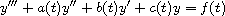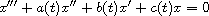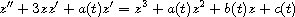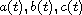Electron. J. Diff. Equ., Vol. 2010(2010), No. 174, pp. 1-10.

### Necessary and sufficient conditions for the oscillation a third-order differential equation Pitambar Das, Jitendra Kumar Pati

Abstract:
We show that under certain restrictions the following three conditions are equivalent: The equationis oscillatory. The equationis oscillatory. The second-order Riccati equationdoes not admit a non-oscillatory solution that is eventually positive.
Furthermore, we obtain sufficient conditions for the above statements to hold, in terms of the coefficients. These conditions are sharp in the sense that they are both necessary and sufficient when the coefficientsare constant.

Submitted April 13, 2010. Published December 6, 2010.
Math Subject Classifications: 34C10, 34C15.
Key Words: Oscillation; non-oscillation; third order differential equations.

Show me the PDF file (224 KB), TEX file, and other files for this article.Pitambar Das Department of Mathematics, Indira Gandhi Institute of Technology Sarang-759146, Talcher, Orissa, India email: pdasigit@in.com Jitendra Kumar Pati Department of Mathematics, Indira Gandhi Institute of Technology Sarang-759146, Talcher, Orissa, India email: jkpati2007@yahoo.com